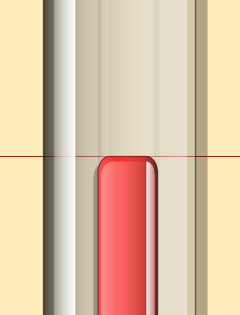# Celsius-Fahrenheit Converter

 °C to °F °F to °C °C °FFour ways to convert from Celsius to Fahrenheit:
• Divide by 5, multiply by 9, then add 32.
• Multiply by 18, divide by 10, then add 32.
• Multiply by 10, subtract original temperature, divide by 5, then add 32.
• Multiply by 10, subtract original temperature, double it, divide by 10, then add 32.
So, converting 10°C using the first formula: 10/5=2, 2x9=18, 18+32=50°F
Three ways to convert from Fahrenheit to Celsius:
• Subtract 32, divide by 9, then multiply by 5.
• Subtract 32, divide by 3, multiply by 5, divide by 3.
• Subtract 32, divide by 3, multiply by 10, divide by 6.
So, converting 212°F using the first formula: 212-32=180, 180/9=20, 20x5=100°C
To convert from Celsius to Kelvin (absolute):
• Subtract 273.15
To convert from Kelvin (absolute) to Celsius: